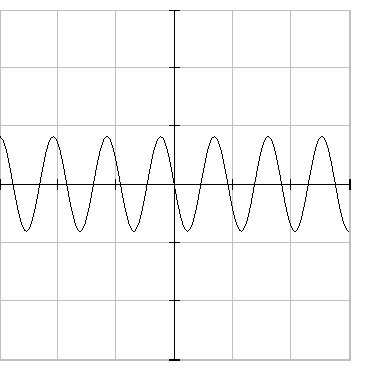number of oscillations = 5.5
total time = number of divisions * time per division = 8 * 0.001 = 0.008 s
frequency = number of oscillations / total time = 5.5 / 0.008 = 688 Hz

y(x) = 6.54*sin(2*pi*(5.5(x--50)/100+0.25))
number of oscillations =
number of divisions =
time per division = unit for time per division =
left phase =

grid on grid off
x-axis variable = x-axis unit = x-axis min = x-axis max =
y-axis variable = y-axis unit = y-axis min = y-axis max =
graph width in pixels = graph height in pixels =